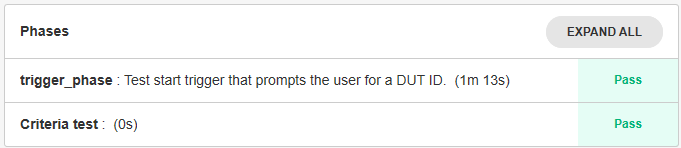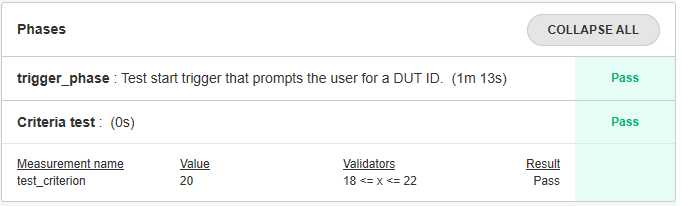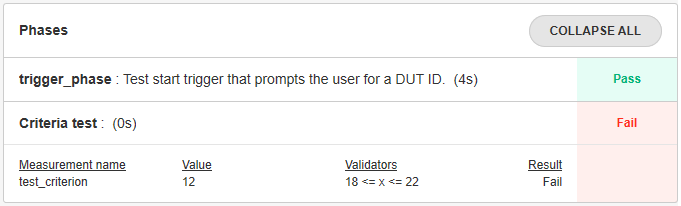# 9. Test Criteria Tutorial¶

## Defining Test Criteria¶

The criteria refer to the thresholds against which measures are compared to declare a test case PASS or FAIL. In the spintop-openhtf context, the measures module implements the criteria and the comparison against the selected values.

To define a test criterion, first define an openhtf measurement object.

```import openhtf as htf
criterion = htf.Measurement('test_criterion').in_range(18, 22)
```

Here the criterion defined will return a PASS if the value evaluated is between 18 and 22. The criterion name is “test_criterion” and it has been stored in the criterion variable.

Use the htf.measures decorator to use the defined criterion in a test case.

```@plan.testcase('Criteria test')
@htf.measures(criterion)
def criteria_test(test):
value = 20
test.measurements.test_criterion =  value
```

The criterion object is loaded in the openhtf measures. To evaluate a value against the test criterion, simply equate the value to the criterion. Note that the criterion name (“test_criterion”) is used and not the object variable.

Add the defined criteria and test case to your latest test bench and run it. You will see that the new test phase called Criteria test has passed.Hit expand all on the Phases box and see that the evaluated criteria has been added to the test phase result.Modify the value in the test case code to use a value outside of the criteria range.

```@plan.testcase('Criteria test')
@htf.measures(criterion)
def criteria_test(test):
value = *12*
test.measurements.test_criterion = value
```

Run the test bench again. The phase will now fail.`Tutorial source`

## Criteria types¶

In the example above, a range was defined to instanciate the criteria. Multiple different validator types can be used instead of the range function.

A good practice is to use a validator function which will return True or False depending on the value evaluated. For example, our range criteria can be defined in another manner as

```def range_validator(value):
return 18 =< value =< 22

criterion = htf.Measurement('test_criterion').with_validator(range_validator)
```

However, doing so will remove the pretty-printing of the validator. For example, the built-in `in_range` validator will print out as “{min} < x < {max}”.

Using the with_validator function helps you create complex criteria that match your exact needs.

For more details on the different types of criteria that can be implemented please refer to the Measurements reference

## Documentation¶

It is possible to add documentation to the criterion with the doc() function

```criterion = htf.Measurement('test_criterion').in_range(18, 22)
.doc('This measurement helps illustrate the criteria usage in spintop-openhtf')
```

## Using a criteria definition file¶

As we have seen in the 6. Proposed Project Structure tutorial , we believe it is a good practice to seperate the criteria definition from the actual test logic of the test bench. This guideline is proposed because it allows the modification and the tracking of all criteria in a single emplacement. It also eliminates the duplication of criteria.

To this purpose, we propose to create a file called, for example, criteria.py. In this file a function called get_criteria() takes the criterion name as argument and returns the criterion which the @htf.measures decorator uses.

Create the file and implement the function as described below.

```import openhtf as htf

def get_criteria(criterion):
criteria_dict = {
"test_criterion":    htf.Measurement('test_criterion').in_range(18,22)
}

return criteria_dict[criterion]
```

The python dictionary acts as a criteria switch case. The function selects the required measurement object and returns it to the caller.

In your test bench, use the function in the @htf.measures decorator to load the criteria and use it directly.

```from criteria import get_criteria

@plan.testcase('Criteria test')
@htf.measures(get_criteria('test_criterion'))
def criteria_test(test):
value = 20
test.measurements.test_criterion = value
```

Run the test bench again and the result should be the same as was obtained above.

`Tutorial source`

`Criteria file`

## Dynamic Test Criteria¶

Test criteria can be defined dynamically in the test logic. Two major reasons do so are:

• To create criteria based on the product definition configuration

• To create criteria based on previous results or measurements

### Defining a Dynamic Test Criterion¶

To define a dynamic criterion, use the htf.Measurement function as in the static definitions, but instead as defining it as a function decorator, create a new entry in the state.running_phase_state.measurements dictionary. Also, the test case must have access to the state object. To do so,

• Add the requires_state=True attribute to the testcase defintion decorater

• Instead of passing the test variable to the test case function, the state variable must be passed.

• For the evaluation of the test criterion, the measurements dictionary must be accessed from the state object instead of the test objects (state.test_api.measurements)

```@plan.testcase('Dynamic Criterion Test', requires_state=True)
def criteria_test(state):
value = 12
state.running_phase_state.measurements['VOLTAGE'] = htf.Measurement('VOLTAGE').
in_range(11.5,12.5)
state.test_api.measurements.VOLTAGE = value
```

### Based on a product defintion¶

A good example of a criterion based on a product definition is a criterion defined around the input voltage of a device. For example, the product defintion states that the input voltage is 12V. A criterion defined around the voltage would state that a measure of 12V +/- 0.5 Volts would indicate a PASS.

First create a file name product.py, and implement the voltage definition within it.

```class voltage():
input_voltage = 12
```

Then define the dynamic criterion importing the value from the product definition.

```from product import voltage

@plan.testcase('Dynamic Criterion Test from Product Definition', requires_state=True)
def product_definition_test(state):

#value = measure_input_voltage()
value = 12

#definition of criterion
state.running_phase_state.measurements['VOLTAGE'] = htf.Measurement('VOLTAGE').
in_range(voltage.input_voltage - 0.5, voltage.input_voltage + 0.5)

#evaluation of criterion
state.test_api.measurements.VOLTAGE = value
```

### Based on a previous measurement¶

The same method can be used to define a criterion from a previous measurement. For example, testing a voltage divider, which has been set to produce a voltage 30% of the input voltage. The input voltage has been measured as 12.05 volts. To create the divided voltage criterion, the measured value must be used in its definition, not the nominal value.

```@plan.testcase('Dynamic Criterion Test from Previous Measurement', requires_state=True)
def previous_measurement_test(state):

#measured_input = measure_input_voltage()
measured_input = 12.05

#divider_voltage = measure_divider_voltage()
divider_voltage = 3.55

#definition of criterion as within +/- 5% of the divider voltage, which is 30% on the measured input
state.running_phase_state.measurements['DIVIDER_VOLTAGE'] = htf.Measurement('DIVIDER_VOLTAGE').
in_range(measured_input * 0.3 * 0.95, measured_input * 0.3 * 1.05)

#evaluation of criterion
state.test_api.measurements.DIVIDER_VOLTAGE = divider_voltage
```

`Tutorial source`

`Product Definition file`

## OpenHTF Validators¶

Module containing canned measurement validators.

Additional validators may be registered by passing them to the Register() method. They can then be accessed directly as attributes on the validators module, and will typically be a type, instances of which are callable:

from openhtf.util import validators from openhtf.util import measurements

class MyLessThanValidator(ValidatorBase):
def __init__(self, limit):

self.limit = limit

# This will be invoked to test if the measurement is ‘PASS’ or ‘FAIL’. def __call__(self, value):

return value < self.limit

# Name defaults to the validator’s __name__ attribute unless overridden. validators.register(MyLessThanValidator, name=’LessThan’)

# Now you can refer to the validator by name directly on measurements. @measurements.measures(

measurements.Measurement(‘my_measurement’).LessThan(4))

def MyPhase(test):

test.measurements.my_measurement = 5 # Will have outcome ‘FAIL’

If implemented as a class, inherit from a suitable base class defined in this module; such validators may have specialized handling by the infrastructure that you can leverage.

For simpler validators, you don’t need to register them at all, you can simply attach them to the Measurement with the .with_validator() method:

def LessThan4(value):

return value < 4

@measurements.measures(

measurements.Measurement(‘my_measurement).with_validator(LessThan4))

def MyPhase(test):

test.measurements.my_measurement = 5 # Will also ‘FAIL’

Notes:

Note the extra level of indirection when registering a validator. This allows you to parameterize your validator (like in the LessThan example) when it is being applied to the measurement. If you don’t need this level of indirection, it’s recommended that you simply use .with_validator() instead.

Also note the validator will be str()’d in the output, so if you want a meaningful description of what it does, you should implement a __str__ method.

Validators must also be deepcopy()’able, and may need to implement __deepcopy__ if they are implemented by a class that has internal state that is not copyable by the default copy.deepcopy().

class `openhtf.util.validators.``Equals`(expected, type=None)

Validator to verify an object is equal to the expected value.

class `openhtf.util.validators.``InRange`(minimum=None, maximum=None, type=None)

Validator to verify a numeric value is within a range.

property `maximum`

Should return the maximum, inclusive value of the range.

property `minimum`

Should return the minimum, inclusive value of the range.

class `openhtf.util.validators.``RegexMatcher`(regex, compiled_regex)

Validator to verify a string value matches a regex.

class `openhtf.util.validators.``WithinPercent`(expected, percent)

Validates that a number is within percent of a value.

property `maximum`

Should return the maximum, inclusive value of the range.

property `minimum`

Should return the minimum, inclusive value of the range.

`openhtf.util.validators.``in_range`